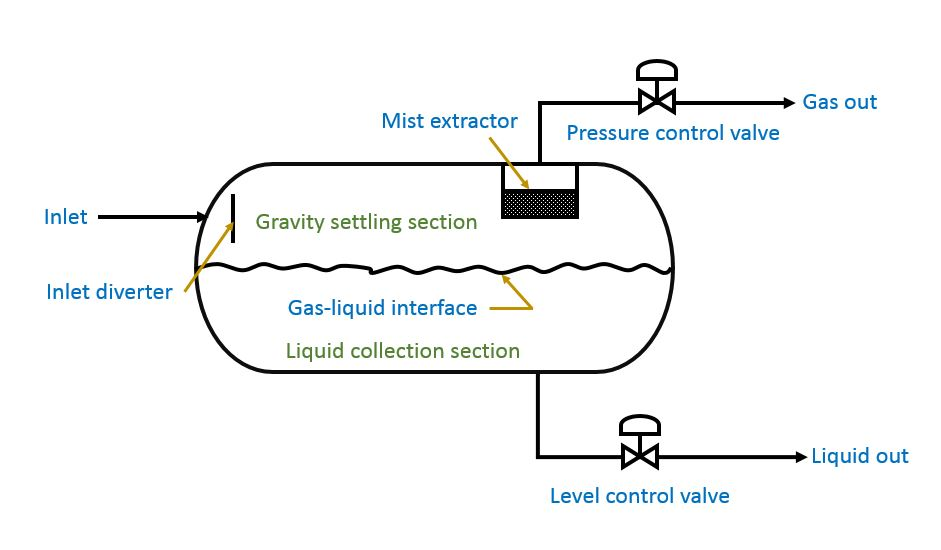# Preliminary Sizing of Two-Phase Horizontal Separator

Separator is the initial processing vessel in any production facility. Therefore, it should be designed properly to prevent reduction of capacity of the entire facility.

Separators are classified into two-phase and three-phase separator. Two-phase separators are used to separate gas from the total liquid stream. Meanwhile, three-phase separators are used to separate gas from crude oil and water component.

Separators are designed in either horizontal, vertical, or spherical configuration. In this post, I want to share to you how to size two-phase horizontal separator.

Schematic of Two-Phase Horizontal Separator

Figure below is schematic drawing of two-phase horizontal separator.

Initial bulk separation of gas and liquid takes place in inlet diverter. The fluid mixture hits inlet diverter causing sudden change in momentum, because of the gravity difference, results in bulk separation of gas from liquid. The liquid droplet fall out of the gas stream to the bottom of vessel where it is collected.

The liquid collection section provides retention time to let entrained gas evolve out of the liquid and rise to vapor space. If required, it also provides surge volume to handle intermittent slugs of liquid.

The liquid leaves the separators through the liquid control valve. The valve is regulated by a level controller. The level controller senses changes in liquid level and controls the valve accordingly.

The gas flow through the gravity settling section above the liquid. In this section, small drop of liquid that were entrained in the gas and not separated by the inlet diverter are separated by gravity and fall to the gas-liquid interface. Some of the droplet are too small in diameter, so that it cannot be easily separated in the gravity settling section. Before the gas leaves the separator, it passes through coalescing section or mist extractor. This section uses element of vanes, wire mesh, or plates to coalesce and remove very small droplets of liquid in one final separation before the gas leaves the vessel.

Pressure in the separator is maintained by a pressure controller. The pressure controller senses changes in pressure and sends a signal to open or close the pressure control valve. By controlling the rate at which gas leaves the vapor space of the vessel, the pressure in the vessel is maintained.

Step-by-Step Sizing of Two-Phase Horizontal Separator

Figure below shows step-by-step sizing of two-phase horizontal separator.

I will use an example to explain step-by-step sizing of two-phase horizontal separator.

Example of Two-Phase Horizontal Separator Sizing

Determine the diameter and height (seam-to-seam length) of a two-phase horizontal separator for the following operating condition:

Gas rate:                              10 MMscfd

Gas specific gravity:            0.6

Oil rate:                               2000 BOPD (barrel oil per day)

Oil gravity:                          40o API

Operating pressure:           1000 psia

Operating temperature:     60oF

Let’s start sizing of two-phase separator.

Step 1: Collect the Data

Gas rate, gas specific gravity, oil rate, oil gravity, operating pressure, and operating temperature are already defined above.

Step 2: Find Other Properties (Z factor, gas viscosity)

For 0.6 gas specific gravity, 1000 psia operating pressure, and 60oF operating temperature, we get Z factor 0.83. Use this figure to find Z factor for gas specific gravity 0.6.

For 0.6 gas specific gravity, 1000 psia operating pressure, and 60oF operating temperature, we get gas viscosity 0.013 cP. Use this figure to find gas viscosity.

Step 3: Calculate K (Constant used in gas capacity equation)

K is obtained from figure below.

K is a function of S P/T and oil API.

Where:

S = specific gravity of of gas capacity

P = pressure (psia)

T = temperature (oR)

From the case above,

S P/T = 0.6 x 1000 / (60 + 460)

S P/T = 1.154

For S P/T 1.154 and oil gravity of 40o API, we get K value of 0.28.

Step 4: Calculate Gas Capacity Constraint (d Leff)

Gas capacity constraint is calculated by using the following equation.

We get gas capacity constraint (d Leff) is 50.76 in.ft.

Step 5: Calculate seam-to-seam length (Lss) for various separator diameter (d)

Seam-to-seam length (Lss) for gas capacity constraint is calculated by using the following equation.

Seam-to-seam length for various separator diameter is shown in table below.

Step 6: Calculate liquid capacity constraint (d2Leff)

Liquid capacity constraint is calculated by using the following equation.

Step 7: Calculate combinations of diameter (d) and seam-to-seam length (Lss) for various retention time (tr)

Seam-to-seam length (Lss) for liquid capacity constraint is calculated by using the following equation.

Table below shows combination of separator diameter and length for liquid capacity constraint.

Step 8: Calculate slenderness ratio (12 Lss/d). Choose between 3 or 4

See step 7.

Step 9: Graph results and choose reasonable size with a diameter and length combination above both gas capacity and liquid capacity constraint line

Graph below show vessel internal diameter and vessel length for various retention time.

Free Spreadsheet for Two-Phase Horizontal Separator

I hope you find this post useful.

References:

• Surface Production Operation Vol. 1: Design of Oil-Handling Systems and Facilities
• GPSA Engineering Data Book